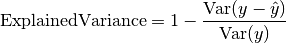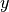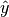Shortcuts

# Explained Variance¶

## Module Interface¶

class torchmetrics.ExplainedVariance(multioutput='uniform_average', **kwargs)[source]

Computes explained variance:Whereis a tensor of target values, andis a tensor of predictions.

As input to forward and update the metric accepts the following input:

• preds (Tensor): Predictions from model in float tensor with shape (N,) or (N, ...) (multioutput)

• target (Tensor): Ground truth values in long tensor with shape (N,) or (N, ...) (multioutput)

As output of forward and compute the metric returns the following output:

In the case of multioutput, as default the variances will be uniformly averaged over the additional dimensions. Please see argument multioutput for changing this behavior.

Parameters
• multioutput (str) –

Defines aggregation in the case of multiple output scores. Can be one of the following strings (default is 'uniform_average'.):

• 'raw_values' returns full set of scores

• 'uniform_average' scores are uniformly averaged

• 'variance_weighted' scores are weighted by their individual variances

• kwargs (Any) – Additional keyword arguments, see Advanced metric settings for more info.

Raises

ValueError – If multioutput is not one of "raw_values", "uniform_average" or "variance_weighted".

Example

>>> from torchmetrics import ExplainedVariance
>>> target = torch.tensor([3, -0.5, 2, 7])
>>> preds = torch.tensor([2.5, 0.0, 2, 8])
>>> explained_variance = ExplainedVariance()
>>> explained_variance(preds, target)
tensor(0.9572)

>>> target = torch.tensor([[0.5, 1], [-1, 1], [7, -6]])
>>> preds = torch.tensor([[0, 2], [-1, 2], [8, -5]])
>>> explained_variance = ExplainedVariance(multioutput='raw_values')
>>> explained_variance(preds, target)
tensor([0.9677, 1.0000])


Initializes internal Module state, shared by both nn.Module and ScriptModule.

## Functional Interface¶

torchmetrics.functional.explained_variance(preds, target, multioutput='uniform_average')[source]

Computes explained variance.

Parameters
• preds (Tensor) – estimated labels

• target (Tensor) – ground truth labels

• multioutput (str) –

Defines aggregation in the case of multiple output scores. Can be one of the following strings):

• 'raw_values' returns full set of scores

• 'uniform_average' scores are uniformly averaged

• 'variance_weighted' scores are weighted by their individual variances

Example

>>> from torchmetrics.functional import explained_variance
>>> target = torch.tensor([3, -0.5, 2, 7])
>>> preds = torch.tensor([2.5, 0.0, 2, 8])
>>> explained_variance(preds, target)
tensor(0.9572)

>>> target = torch.tensor([[0.5, 1], [-1, 1], [7, -6]])
>>> preds = torch.tensor([[0, 2], [-1, 2], [8, -5]])
>>> explained_variance(preds, target, multioutput='raw_values')
tensor([0.9677, 1.0000])

Return type

© Copyright Copyright (c) 2020-2023, Lightning-AI et al... Revision 825d17f3.

Built with Sphinx using a theme provided by Read the Docs.
Versions
latest
stable
v0.11.3
v0.11.2
v0.11.1
v0.11.0
v0.10.3
v0.10.2
v0.10.1
v0.10.0
v0.9.3
v0.9.2
v0.9.1
v0.9.0
v0.8.2
v0.8.1
v0.8.0
v0.7.3
v0.7.2
v0.7.1
v0.7.0
v0.6.2
v0.6.1
v0.6.0
v0.5.1
v0.5.0
v0.4.1
v0.4.0
v0.3.2
v0.3.1
v0.3.0
v0.2.0
v0.1.0